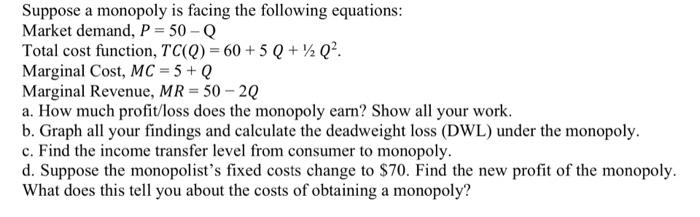Home / Expert Answers / Economics / suppose-a-monopoly-is-facing-the-following-equations-market-demand-p-50q-total-cost-function-pa703

# (Solved): Suppose a monopoly is facing the following equations: Market demand, P=50Q Total cost function, ...Suppose a monopoly is facing the following equations: Market demand, Total cost function, . Marginal Cost, Marginal Revenue, a. How much profit/loss does the monopoly earn? Show all your work. b. Graph all your findings and calculate the deadweight loss (DWL) under the monopoly. c. Find the income transfer level from consumer to monopoly. d. Suppose the monopolist's fixed costs change to . Find the new profit of the monopoly. What does this tell you about the costs of obtaining a monopoly?

We have an Answer from Expert

a. To determine the profit/loss earned by the monopoly, we need to find the quantity at which marginal revenue (MR) equals marginal cost (MC). At this quantity, the monopoly maximizes its profit.

Given:
Market demand: P = 50 - Q
Total cost function: TC(Q) = 60 + 5Q + ½Q²
Marginal Cost: MC = 5 + Q
Marginal Revenue: MR = 50 - 2Q

To find the profit-maximizing quantity, we equate MR and MC:
50 - 2Q = 5 + Q

Simplifying the equation, we get:
3Q = 45
Q = 15
We have an Answer from Expert